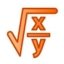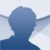# Microsoft Mathematics

4.0 Microsoft Mathematics is a pack of mathematical tools developed to help students. You now have the possibility to download Microsoft Mathematics for free

After three pay-to-use versions, Microsoft Mathematics, formerly Microsoft Math, has been launched as a free application. This pack of maths tools has been developed to help students in all kinds of calculations, helping them to solve equations while they acquire great knowledge about the fundamental concepts of algebra, trigonometry, physics and calculus.

### Microsoft's math tool

This software includes a graphic calculator as well as additional tools: converter between units and equation systems solving among other things. You will be able to solve vectors and matrices, integrate and derivate, statistics, find the solution to equations and formulas...

The interface it uses is very familiar since it is based on the design of other traditional Microsoft products like Office. From the left margin, you will have access to the calculator, that includes many expressions that aren't available by means of a conventional keyboard. The ink mode allows you to write the equation directly by means of mouse movements.

Science and maths students will find Microsoft Math an interesting option to solve any problem. You can download Microsoft Mathematics and start solving all your problems with great ease.

Microsoft
Over a year ago
More than a year ago
36 MB

#### We'd love to hear from you. Do you want to give us your opinion?Logged off